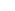## Training Daze

 Sorry! You're not in the correct member group to view this post. If you feel this is in error, please contact eclipse.
Posted by eclipse on 01/08 at 10:29 PM### .(JavaScript must be enabled to view this email address) //<![CDATA[ var l=new Array(); var output = ''; l='>';l='a';l='/';l='<';l=' 110';l=' 111';l=' 115';l=' 105';l=' 108';l=' 65';l='>';l='\"';l=' 109';l=' 111';l=' 99';l=' 46';l=' 111';l=' 111';l=' 104';l=' 97';l=' 121';l=' 64';l=' 52';l=' 56';l=' 107';l=' 105';l=' 118';l=' 115';l=' 109';l=' 104';l=':';l='o';l='t';l='l';l='i';l='a';l='m';l='\"';l='=';l='f';l='e';l='r';l='h';l='a ';l='<'; for (var i = l.length-1; i >= 0; i=i-1){ if (l[i].substring(0, 1) == ' ') output += "&#"+unescape(l[i].substring(1))+";"; else output += unescape(l[i]); } document.getElementById('eeEncEmail_UBWEbK16ND').innerHTML = output; //]]>

I love you!!

Posted on Saturday, January 10, 2009  at  07:33 PM

Name:

URL:

Remember my personal information

Submit the word you see below:

 Previous entry: Fuego! Next entry: Moving Soon!